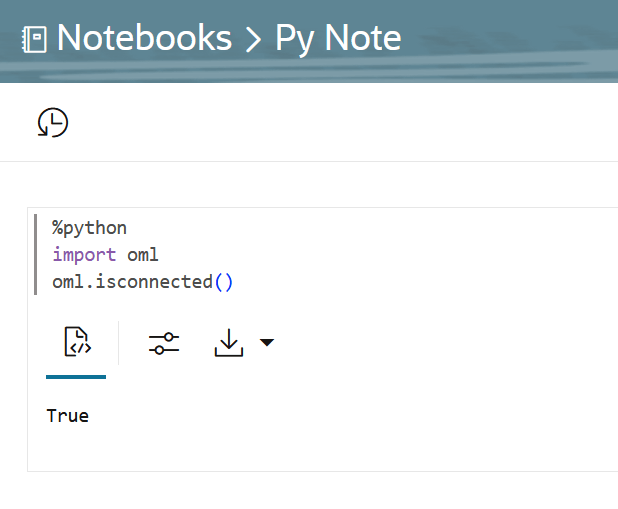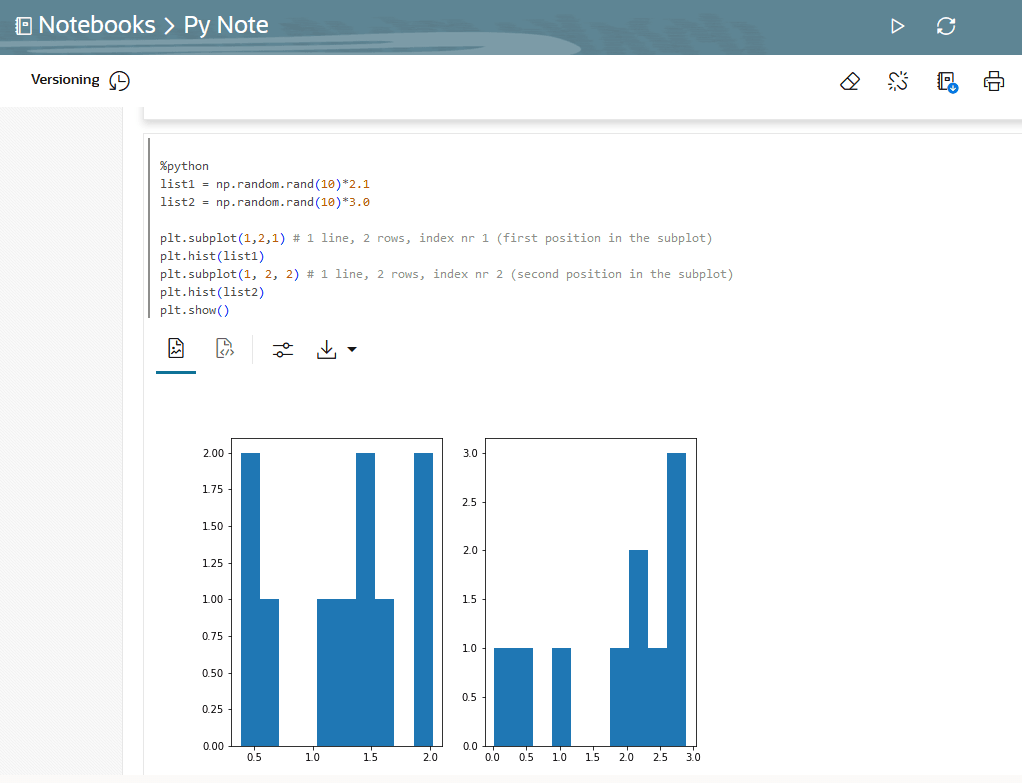## 4.3.2 Run a Notebook with Python Interpreter

To run Python commands in a notebook, you must first connect to the Python interpreter. To use OML4Py, you must import the `oml` module.

In an Oracle Machine Learning notebook, you can add multiple paragraphs, and each paragraph can be connected to different interpreters such as SQL or Python. This example shows you how to:
• Connect to a Python interpreter to run Python commands in a notebook
• Import the Python modules - `oml`, `matplotlib`, and `numpy`
• Check if the `oml` module is connected to the Oracle Database

Note:

`z` is a reserved keyword and must not be used as a variable in `%python` paragraphs in Oracle Machine Learning Notebooks.
Assumption: The example assumes that you have created a new notebook named Py Note.
1. Open the Py Note notebook and click the interpreter bindings icon. View the available interpreter bindings.
2. To connect to the Python interpreter, type `%python`
You are now ready to run Python scripts in your notebook.
3. To use OML4Py module, you must import the `oml` module. Type the following Python command to import the `oml` module, and the click run icon. Alternatively, you can press Shift+Enter keys to run the notebook.
`import oml`
4. To verify if the `oml `module is connected to the Database, type:
`oml.isconnected()`Description of the illustration connect_python.eps
Once your notebook is connected, the command returns `TRUE.` The notebook is now connected to the Python interpreter, and you are ready to run python commands in your notebook.

Example to demonstrate the use of the Python modules - `matplotlib` and `numpy` , and use random data to plot two histograms.

1. Type the following commands to import the modules:
``````%python
import matplotlib.pyplot as plt
import numpy as np``````
• `Matplotlib` - Python module to render graphs
• `Numpy` - Python module for computations
2. Type the following commands to compute and render the data in two histograms.
``````
list1 = np.random.rand(10)*2.1
list2 = np.random.rand(10)*3.0

plt.subplot(1,2,1) # 1 line, 2 rows, index nr 1 (first position in the subplot)
plt.hist(list1)
plt.subplot(1, 2, 2) # 1 line, 2 rows, index nr 2 (second position in the subplot)
plt.hist(list2)
plt.show()``````
In this example, the commands import two Python module to compute and render the data in two histograms list1 and list2.
3. Click Run.Description of the illustration python-script.eps
The output section of the paragraph which contains a charting component displays the results in two histograms - list1 and list2, as shown in the screenshot.